Embedded Programming Group Assignment

For this section I will compare the performance of 3 boards corresponding to the ATtiny1614, Arduino UNO (ATMEGA328P), Arduino DUE (AT91SAM3X8E).

The code used is the same for all tests.

/*
* pi.ino
* Neil Gershenfeld 12/20/20
* pi calculation benchmark
* pi = 3.14159265358979323846
*/

#define NPTS 100000

float a,b,c,pi,dt,mflops;
unsigned long i,tstart,tend;

void setup() {
Serial.begin(115200);
}

void loop() {
tstart = millis();
a = 0.5;
b = 0.75;
c = 0.25;
pi = 0;
for (i = 1; i <= NPTS; ++i)
pi += a/((i-b)*(i-c));
tend = millis();
dt = (tend-tstart)/1000.0;
mflops = NPTS*5.0/(dt*1e6);
Serial.print("NPTS = ");
Serial.print(NPTS);
Serial.print(" pi = ");
Serial.println(pi);
Serial.print("time = ");
Serial.print(dt);
Serial.print(" estimated MFlops = ");
Serial.println(mflops);
}

For the ATtiny1614 board the performance is as follows: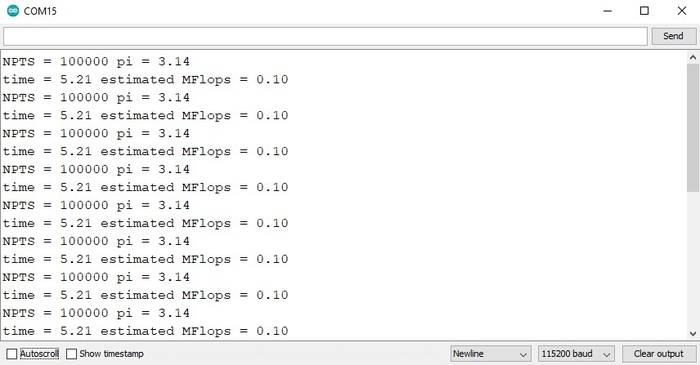If we compare it with the previous generation (ATMEGA328P) we can see that the ATtiny1614 has an improvement in performance.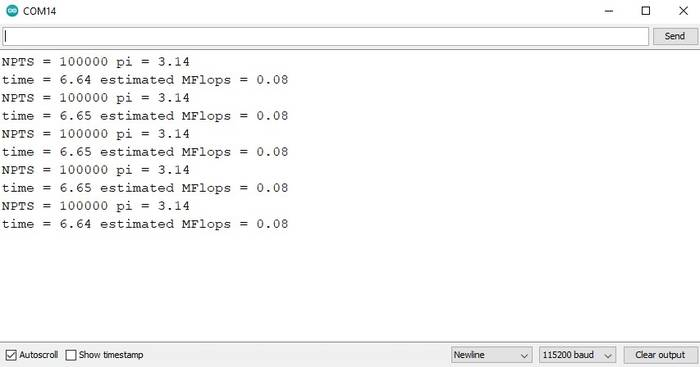For the last test I used an Arduino DUE (AT91SAM3X8E) with a 32-bit ARM architecture. By default the library to program this board is not included in the Arduino IDE but it can be added very easily.

In the Arduino IDE go to the Tools -> Board: ... -> Boards Manager ... option.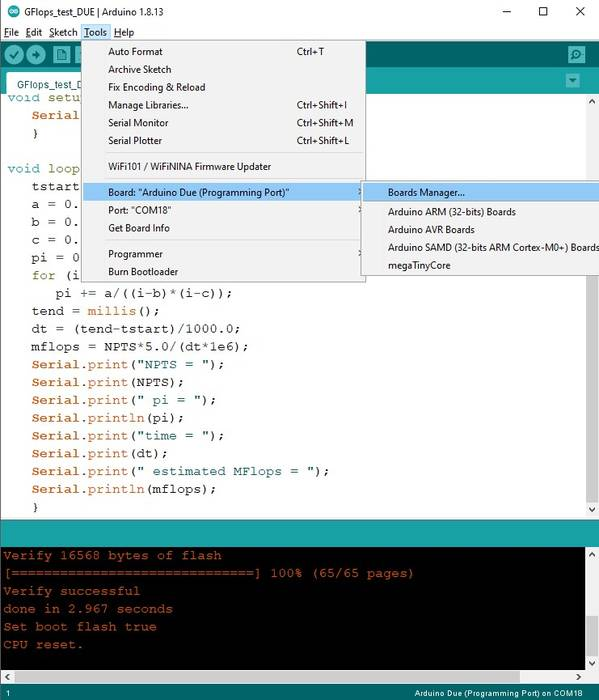Then in the search bar type "Arduino DUE" and the following result will appear, just click on install and that's it.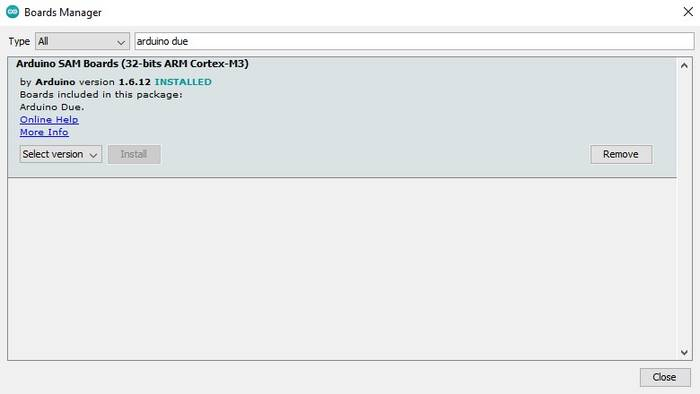The result for the Arduino DUE board is as follows.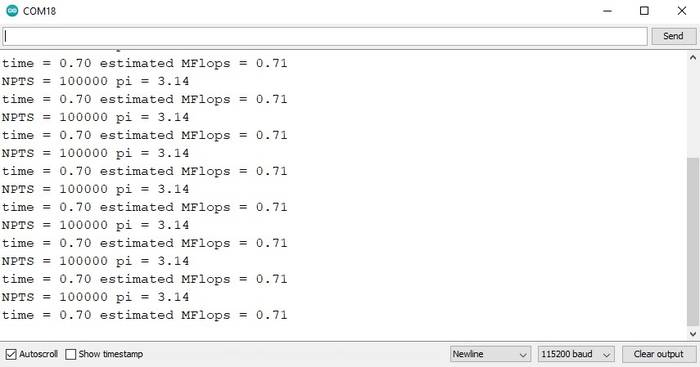Indeed, it has better performance than the previous boards.